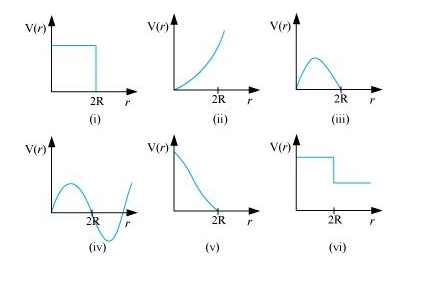# Which of the following potential energy curves in Fig. 6.18 cannot possibly

Question.
Which of the following potential energy curves in Fig. 6.18 cannot possibly describe the elastic collision of two billiard balls? Here r is the distance between centres of the balls.solution:

(i), (ii), (iii), (iv), and (vi)

The potential energy of a system of two masses is inversely proportional to the separation between them. In the given case, the potential energy of the system of the two balls will decrease as they come closer to each other. It will become zero $($ i.e., $V(r)=0)$ when the two balls touch each other, i.e., at $r=2 R$, where $R$ is the radius of each billiard ball. The potential energy curves given in figures (i), (ii), (iii), (iv), and (vi) do not satisfy these two conditions. Hence, they do not describe the elastic collisions between them.